# The ROBUSTREG Procedure

### Implementation of the WEIGHT Statement

Subsections:

You can use the WEIGHT statement to specify a weight variable in the input data set. (For more information, see the section WEIGHT Statement.) This section describes how PROC ROBUSTREG implements the WEIGHT statement for each of the estimation methods and for leverage detection.

#### M Estimation

If you use M estimation with a known scale, then instead of minimizing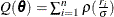, the weighted M estimation minimizes the weighted Huber-type objective function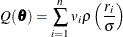where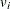is the weight variable that is specified by the WEIGHT statement. If you use M estimation with an unknown scale, the weight variable is used in the location steps but not in the scale steps. (For more information, see the section M Estimation and the SCALE= option.) For estimating the covariance of the weighted M estimation,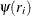and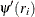are obtained from the final iteration of the weighted M estimation, and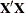and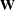are replaced, respectively, by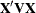and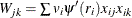, where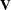is a diagonal matrix whose diagonal elements are. (For more information, see the section Asymptotic Covariance and Confidence Intervals.) The weight variable does not affect the model degrees of freedom p and the error degrees of freedom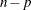.

#### LTS Estimation

LTS estimation ignores the weight variable.

#### S Estimation

S estimation applies the weight variable only in its M-refinement step. Except for the initial estimates, the M-refinement step of S estimation is the same as the weighted M estimation with unknown scale. If you use the NOREFINE suboption, S estimation ignores the weight variable along with the M-refinement step.

#### MM Estimation

By default, the initial step of MM estimation is the initial LTS estimation. Unlike the regular LTS estimation, the initial LTS estimation is applied to the weighted data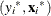’s, where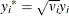and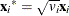. After the initial LTS estimation, the weight variable is ignored for the subsequent scale adjustment.

You can use INITEST=S to specify the initial S estimation as the initial step of the MM estimation. As with the regular S estimation, the weight variable is used only in the M-refinement step of the initial S estimation. There is no subsequent scale adjustment step if the initial S estimation is applied.

Except for the initial estimates, the final M estimation of the MM estimation is the same as the weighted M estimation with known scale.

#### Final Weighted Least Squares Estimation

Final weighted least squares estimation is always applied to the weighted data, no matter how the weight variable is applied in the preceding estimation. For example, if the option METHOD=LTS is specified along with the FWLS option, although the outliers that are identified by LTS estimation do not depend on the weight variable, final weighted least squares estimation applies the weight variable to all the points that are not outliers.

#### Robust Distances and Leverage Detection

Robust distance computation ignores the weight variable. Because leverage detection depends on robust distance, it also ignores the weight variable.